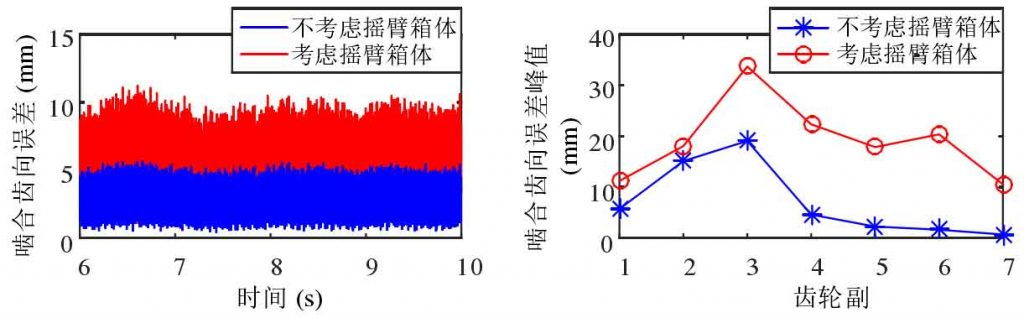# Tooth direction error of multi-stage gear meshing in shearer cutting part

Under the influence of the elastic deformation of the supporting system, the axes of the parallel gears are skewed in the ideal state, which leads to the uneven distribution of the load in the direction of the tooth width and accelerates the failure of the gears. Both ISO 6336 and GB / T 3480 use the meshing tooth direction error f β y to express the deviation degree of the actual position of the tooth profile in the direction of tooth width from the theoretical position, which is an important parameter affecting the bearing capacity of the gear, as shown in Figure 1.

The factors that cause the tooth alignment error include the deformation of the box, bearing, gear shaft and gear. At present, a large number of studies regard the box as rigid, ignoring the influence of its deformation on the transmission system; other studies use finite element software to calculate the deformation of the box under statics. But the shearer drum load has the characteristics of heavy load and strong impact, and the rocker arm box is a cantilever structure, ignoring the box deformation or using static deformation are quite different from the actual situation. Therefore, in this study, the instantaneous rotation axis of each gear pair is obtained by dynamic simulation, and then the instantaneous meshing error of each gear pair is calculated by using Timmers formula. The core idea of Timmers formula is to divide the gear axis skew into parallel error and interleaving error, and then project them to the meshing plane respectively and add them linearly.(a) Instantaneous meshing tooth direction error of gear pair 1(b) Peak value of instantaneous meshing tooth direction error of each gear pair

Figure 2 (a) compares the instantaneous meshing tooth direction error curve of the front and rear gear pair 1 of the coupling rocker box, and it can be seen that the difference between them is very significant. Figure 2 (b) compares the peak value of the meshing tooth direction error of each gear pair. It can be seen that the meshing tooth direction error of each gear pair increases obviously after coupling the rocker arm box, and the increase is related to the specific position of the gear pair in the rocker arm box. Figure 3 shows that if the flexibility of rocker box is not considered, the actual eccentric load of gear pair will be underestimated obviously.

In Fig. 3, on the premise of coupling rocker box, the meshing tooth direction error of gear pair 1 is analyzed retrospectively. Figure 3 (a) analyzes the meshing tooth direction error caused by the deformation of rocker arm box from time domain and frequency domain respectively, with the peak value of 5.0 μ m in time domain; the component below 10 Hz in the frequency spectrum is the excitation frequency of roller load, which is forced vibration; 17.25 Hz is the third frequency of coupling system In addition, the meshing error caused by the deformation of the rocker box also contains the meshing frequency component, but its amplitude is small (FM1 in the figure represents the meshing frequency of the first gear of the cutting transmission system).

Fig. 3 (b) analyzes the meshing tooth alignment error caused by bearing deformation in time domain and frequency domain respectively, and its peak value in time domain is 8.5 μ m; the main components in its frequency spectrum are meshing frequencies of all levels and their frequency doubling. Since the multi-stage gear transmission is composed of two-stage fixed shaft gears and two-stage planetary gear train, fm4 in the figure represents the meshing frequency of the fourth stage gear of the cutting transmission system, that is, the meshing frequency of the second stage planetary gear train.